## Biomedical Research

All submissions of the EM system will be redirected to Online Manuscript Submission System. Authors are requested to submit articles directly to Online Manuscript Submission System of respective journal.

- Biomedical Research (2016) Volume 27, Issue 2

## Human life protection in trenches using gas detection system.

 Ambeth Kumar Devadoss* Associate Professor Computer Science and Engineering, Panimalar Engineering College, India Corresponding Author: Ambeth Kumar Devadoss, Computer Science and Engineering, Panimalar Engineering College,, India. Accepted: January 30, 2016
Visit for more related articles at Biomedical Research

### Abstract

Human beings have numerous sensors to sense the state of the environment and actuators to perform different actions. One such vital sensor is the Nostril. The nostrils are essential for life. Hence, coming in contact with hazardous gases causes danger to human life. In many developing countries, lthe sewers are still cleaned by unskilled labourers. Situations may arise where hazardous gas is emitted via sewage and can potentially endanger life. Furthermore, in coal mining, there is a possibility of hitting a source of natural gas which cannot be determined unless or until a sensor is utilized. To prevent such hazardous situations, this new gas detection system detects those types of gases, analyzes them for us and provides essential details about it. Our system is designed to track the presence of hazardous gases, identify the safety limit and calculate the level in that situation, thereby preventing hazards to human life. It allows detection of carbon monoxide and methane at the given time, along with their accurate concentration values in ppm. The basic idea of this system is to calculate a safe limit point 'x', when the system detects a value 'y' (near to and less than 'x') and then alert the environment. If the value exceeds 'x' ,then system should provide a higher level threat detection alert so that the persons immediately evacuate that area, thereby preventing any possible dangers. The alert messages are broadcasted using GSM technology and hence, can be used to notify other rescue workers about the potential hazard the worker is facing at the moment.

### Keywords

Human, Hazards gas, GSM, Environment.

### Introduction

The ardent passion towards contributing to the society in order to save human lives was the main reason behind the origin of this project. Various articles which stated the numerous cessations of human lives while attempting to treat underground sewers intrigued our team to develop the model [1,2]. For those who work in atmospheres that could be hazardous to their health, selecting the right gas detector could be the single most important decision they could ever make. Their life could hinge on that decision so it is critical that the user make him aware of the hazards that could be encountered and the proper sensors to protect them. Data gathered in the late seventies and early eighties indicated that 65% of all those who died in confined spaces were unaware that the space they were entering was a potential hazard. Over 50% of confined space deaths occur to the rescuers and over one third of the fatalities occurred after the space was tested and declared safe and the gas detector was removed.
Gas detectors have been around for a long time, starting with that infamous methane sniffing canary, which sadly was a oneshot device, which when subjected to methane, tended to die rather quickly with no audio and visual alarm capabilities other than being slightly cheap and a total lack of motion. Fortunately technology has advanced significantly and we find ourselves at this point in time with some very sophisticated electronic equipment. But even the most sophisticated technology is useless if the sensors used are unable to detect the gases present.
Such references and may more throw light on the fact that the sewer gases contribute a major role in the mortality rate. The overall causal ratio may be small in comparison with others, but is still not a good reason to ignore the situation as life, however small it may be still has to be valued.
Tragedies like this frequently happen when gas monitors either are used improperly or not used at all. Manufacturers tirelessly work to make their instruments easier to use in an effort to eliminate such tragedies. But no matter how user-friendly the instruments become, if employees do not use them properly, injury and death on the job will continue to occur. Deciding to use a gas detector is a smart thing to do. These factors formed the basis behind the origin of the paper.
Sewer gas is a complex mixture of toxic and nontoxic gases produced and collected in sewage systems by the decomposition of organic household or industrial wastes, typical components of sewage. Sewer gases may include hydrogen sulfide, ammonia, methane, carbon monoxide, sulfur dioxide, and nitrogen oxides. Improper disposal of petroleum products such as gasoline and mineral spirits contribute to sewer gas hazards. Sewer gases are of concern due to their odor, health effects, and potential for creating fire or explosions.
In developing countries like India, the sewers are still being cleaned by unskilled laborers. Situations may arise where the hazardous gases emitted by the sewage endanger their lives. Furthermore, a similar situation may arise during the process of coal mining where the miners may get exposed to such harmful gases, a highly concentrated exposure of which, may even lead to their death. Thus an efficient method to detect the presence of such harmful gases becomes a necessity.
Our prime objectives are:
• To track the presence of hazardous gases, the most threatening amongst them namely carbon monoxide and methane present in trenches.
• To identify the safe limits of these gases and calculate the level in that situation.
• Concentration of these toxic gases present in the air is identified accurately in ppm (parts per million).
• To warn the workers about the potential threats that they might face on account of exposure to such gases above a safe limit by sending the effect of the gas at that level through GSM technology.

### Related work

In 2014, Sunny, et al.  proposed a new feature technique called average slope multiplication to quantify individual gases/odours using dynamic responses of sensor array. The ASM approach is a data pre-processing technique based on multiplying the response and recovery data by the respective average slope. The proposed method thus utilizes the new developed feature ASM in the first stage and the specially designed neural quantifiers in the next subsequent stages. ASM is thus used to increase the accuracy in quantification of gases.
Ozmen and Tekce  presented a system which is made of an array of eight phthalocyanine-coated QCM sensors and an ANN to find the corresponding composition of a gas mixture. The system is tested with the following gas mixtures: (1) ethanol–acetone,(2)ethanol–trichloroethylene, (3) acetone– trichloroethylene. The quartz crystal microbalances (QCM) also called acoustic sensors are widely used for detecting the change of resonant frequency of each sensor as a function of gases.
In 2011, Ravi Kumar, et al.  laid before a new soft computational approach for discrimination of gases. The raw data obtained from the responses of oxygen plasma treated thick film tin oxide sensor array exposed to different gases is subjected to wavelet transform and appropriate coefficients were selected using multi scale principal component analysis (MSPCA). The network was found to identify the gases with a high success rate.
Gulbag A and Temurtas F  put forward an Adaptive Neuro- Fuzzy Inference System (ANFIS) for quantitative identification of individual gas concentrations in their gas mixtures. The Quartz Crystal Microbalance (QCM) type sensors were used as gas sensors. The components in the mixture were quantified by applying the steady state sensor responses from the QCM sensor array as inputs to the feed forward neural networks. Acetone and trichloroethylene were evaluated in this study.
Capone S, et al.  proposed an array of highly sensitive and mechanically stable gas sensors based on different sol-gel fabricated Pd-doped SnO2 nanocrystalline thick films which were used to detect concentrations of the range 0-100 ppm CO, 0-4000 ppm CH4 at 50% relative humidity. The selectivity of the Pd content had been modulated by varying the percentage of the Pd content. Principal Component Analysis as pattern recognition and Principal Component Regression as multi - component analysis method have been used to analyze the mixtures.
Jing L, et al.  applied the principle of electronic nose system in artificial olfactory system which combines gas sensor array and artificial neural network recognition. The research involved experimental configuration method of low concentration gas, input, output and hidden layer element determination method. The system was able to portray lower error rate in quantitative recognition.
In 2013, Sunny, et al.  came up with a new method for classification of gases/odours called average slope multiplication (ASM) using dynamic characteristics of thick film gas sensor array. The ASM approach is a data preprocessing technique based on multiplying the response and recovery data by the respective average slope. ASM reported superior identification of gases than principal component analysis (PCA) technique
In 2013, Khalaf W  pointed out in Sensor array system for gases identification and quantification that a practical electronic nose for simultaneously estimating many kinds of odour classes and concentrations. The multi-input/multi-output function is decomposed into multiple many to one tasks and ensembles are used to implement them one after the another. An ensemble represents a special kind of odour and realizes the relationship between the sensor array responses and the represented odour concentrations. The ensemble with the smallest relative standard deviation (R.S.D) finally gives the label and concentration of an odour sample.
Dae-Silk Lee, et al.  designed a sensor array with nine discrete sensors integrated on a substrate to recognize the species and quantify the amount of explosive gases. The sensors were designed in such a way to produce uniform thermal distribution and a broad sensitivity to low concentrations through the use of nano-sized sensing materials with high surface areas. Methane, propane and butane are some of the common explosive gases.
A review of the pattern analysis of machine olfaction was laid before by Gutierrez-Osuna R  a member of IEEE. Pattern analysis constitutes a critical building block in the development of gas sensor array instruments capable of identifying and measuring volatile compounds. Summary and guidelines for using the most widely used pattern analysis techniques like neural networks, chemometrics were proposed along with research techniques that are at the frontier of sensor based machine olfaction.

### Proposed Method

A gas detecting system is developed to protect the life of trench workers from toxic gases. The system is designed in such a way to detect the presence of carbon monoxide and flammable gases. The most commonly found flammable gas in trenches is methane. The system uses two sensors namely Mq-2 and Mq-7 for the detection of these gases in air. An Arduino board containing an Atmega 328 microcontroller is used for processing. Arduino senses the environment by receiving inputs from the two sensors, and affects its surroundings by controlling the actuators. The actuators used are an alarm and a LED light. The actuators are configured in such a way they light up or give out a warning noise only if the gases present in air exceed the threshold value.
The whole system is developed using the Arduino programming language in the Arduino development environment. A GSM shield is used to alert the user about the presence of toxic gases using text messages. It can also be used to alert co-workers or rescue workers to help the users in case of an emergency. Given below is the basic system’s architecture Figure 1.
The components present in the gas detecting system are:
• Power Supply Unit
• Sensor Unit
• Arduino
• Lcd Display
• Buzzer with driving circuit
• GSM unit

#### Power supply circuit

The power supply units are designed to convert high voltage AC mains electricity to a suitable low voltage supply for electronic circuits and other devices. The power supply unit in the gas detecting system is used to convert the 230V Alternating current from the mains and provide the electrical components with the required regulated 5V direct Current. The sensors, arduino board and the LCD display needs a regulated power supply but the GSM modem contains a inbuilt voltage regulator and hence does not need the power supply unit.
The sensors, arduino board and the LCD display needs a regulated power supply but the GSM modem contains a inbuilt voltage regulator and hence does not need the power supply unit.
In the circuit as seen in Figure 2A, the transformer of 230v/ 15-0-15v is used to perform the step down operation where a 230V AC appears as 15V AC across the secondary winding. The rectifier is used to convert the Alternating Current (AC) to Direct Current (DC) with the use of four diodes. This process is known as rectification. The filtering circuit is used to smoothen out the circuits so that it doesn’t damage even the most sensitive of the circuits. Capacitor is introduced into the circuit to accomplish the filtering process. The voltage regulator is used to maintain a constant voltage level which is being supplied to devices.

#### Sensor circuit

A sensor is a transducer whose purpose is to detect some characteristic of its environment. It detects any change in events or change in quantity and provides an output as an electrical signal. The two metal oxide semiconductors used are MQ-7 for the detection of carbon monoxide and MQ-2 for the detection of flammable gases.
The sensors can work with very less amount of power supply and hence it makes them portable. The sensors contain 6 pins, 4 of them are used to fetch signals and the other two are used for providing heating current. Optimal heating current is needed for the working of the sensor. The sensors are connected to a power supply of 5V which provides the required circuit voltage for the proper functioning of the sensors. The sensor unit detects the change in environment and reports it to the Arduino in the form of electrical signals.
As seen in Figure 2B, the preferred wiring is to connect both 'A' pins together and both 'B' pins together. It is safer and it is assumed that is has more reliable output results. In the picture, the heater is for +5V and is connected to both 'A' pins. This is only possible if the heater needs a fixed +5V voltage. The variable resistor in the picture is the load-resistor and it can be used to determine a good value. A fixed resistor for the loadresistor is used in most cases.

#### Arduino-sensor circuit

Arduino is used for the processing of the electrical signals received from the two sensors. The connections between the Arduino and the sensor board are shown in the figure 2C. The black wire indicates the ground connection and the red wire indicates 5V circuit voltage. The analog output from the sensor is connected to the analog input pin in the Arduino. Similarly the digital output from the sensor is connected to one of the digital input pins.
GND of Arduino to GND of sensor
5V of Arduino to 5Vv of Sensor
Analog O/p of sensor to any one of the analog ports of Arduino
Digital O/p of sensor to any one of the digital pins of Arduino.
The setup function runs only once, after each power up or reset of the Arduino board. After creating a setup() function, the loop() function does precisely what its name suggests, and loops consecutively, allowing the program to change and respond as it runs. Code in the loop() section of the sketch is used to actively control the Arduino board.
The integer values read using the analog read function are converted back to their analog voltages using the arithmetic calculation:
V = 5.0* analog Read(pin) / 1023
In table 1A, the analog voltage is calculated to find the resistance of the sensor at any moment. A voltage divider circuit explains the resistance calculation.

#### Voltage divider circuit

Figure 2D represents the voltage divider circuit. It contains a load resistor R1 of known value and a variable resistor R2 which keeps changing. Voltage division is the result of distributing the input voltage among the components of the divider.
A simple example of a voltage divider is two resistors connected in series with the input voltage applied across the resistor pair and the output voltage emerging from the connection between them. Since the two resistors are connected in series, the current passing through each resistor at every point is equal.
Ampere(R1) = Ampere(R2)
Ohm's law states that the current through a conductor between two points is directly proportional to the potential difference(voltage) across the two points. Introducing the constant of proportionality, the resistance, one arrives at the usual mathematical equation that describes this relationship:
V= IR
So the amount of current passing through resistor R1 can be expressed as:
Ampere(R1) = V1/ R1
Similarly the current passing through the resistor R2 can be calculated in terms of voltage and resistance. The voltage in resistor R2 is V2 which should be the reduction of voltage V1 from the input voltage. In other words the voltage through the resistors R1 and R2 should sum up to the input voltage Vin. Hence the current through the variable resistor R2 can be calculated by
Ampere(R2)= (V2=Vin-V1)/ R2
Since the amount of current passing though resistor R1 is equal to the current passing through resistor R2
V1/R1 = (Vin-V1)/R2
Multiply with R2 on both sides gives:
R2*V1/R1 = (Vin –V1)
Divide by V1 on both sides gives:
R2/R1 =(Vin –V1)/V1
The variable resistance of the gas sensor can be calculated using the formula:
R2 = R1 * (Vin-V1) / V1
Where R2 is the amount of resistance in the variable resistor. Vin the input voltage and V1 is the voltage at load resistance R1 (Table 1B).
The load resistance is used to accept electric current from a source and causes a voltage drop when it is conducting a current. With no load (open-circuited terminals), all of Vin falls across the output. However, the circuit will behave differently if a load is added. The load resistance used in both MQ-2 and MQ-7 sensor is 1 kohm. This gives good selectivity but increasing the load resistance reduces the accuracy of the concentration of the specific gas present in the air at very high levels.

#### Calibration

Calibration is a comparison between measurements-one of known magnitude or correctness made or set with one device and another measurement made in as similar a way as possible with a second device.
The device with the known or assigned correctness is called the standard. The second device is the device being calibrated. To calibrate the sensors a known reference/standard value is used and the known reference value changes according to the type of sensor being used.
Figure 2E depicts the calibration cylinder. The MQ-7 sensor uses 100 ppm of Carbon Monoxide as the known reference value and the MQ-2 sensor uses 1000 ppm of hydrogen as the known reference value. The calibration procedure’s accuracy is based on this reference value and the whole procedure is only as good as the known standard gas, as shown in figures 2F, 2G and table 1C.
Using the resistance values obtained from the sensor and the resistance of the known standard reference value a ratio is calculated to co-relate with the concentration of the gas present in air. The ratio is calculated using the formula R1/R0. Based on the ratio values the concentration can be calculated in ppm using the sensitivity characteristics of each sensor.
Ordinate contains the resistance ratio of the sensor (R1/R0). Abscissa is the concentration of gases in ppm. R1 is the resistance of gases in different concentrations and Ro is the resistance of sensor in the known standard reference 1000 ppm of Hydrogen. Both the axes are of logarithmic values. The power series equation =4201.7x^-2.692 for the chart is obtained using the formula
Equation: y=c*x^b
c= EXP(INDEX(LINEST(LN(y),LN(x),,),1,2))
b = INDEX(LINEST(LN(y),LN(x),,),1)
The LINEST function calculates the statistics for a line by using the least squares method to calculate a straight line that best fits the data, and then returns an array that describes the line. LINEST function can be combined with other functions to calculate the statistics for other types of models that are linear in the unknown parameters, including polynomial, logarithmic, exponential, and power series (Table 1D). The syntax of LINEST function is
LINEST (known_y's, [known_x's], [const], [stats])
known_y's -An array of y-axis values.
[known_x’s]-An optional argument providing an array of known x-axis values. If provided the known_x’s array should have the same number of values as the known_y’s array. If omitted the array takes on the default values {1,2,3…}
[const]-An optional logical argument that determines how the constant 'b' is treated in the power-line equation y =mx+b. This argument can have the value TRUE or FALSE, If TRUE (or omitted) the constant b is treated normally else if FALSE the constant b is set to 0 in the equation.
[stats] -An optional logical argument which specifies whether or not the function should return additional regression statistics on the line of best fit. This argument can have the value TRUE or FALSE, If FALSE (or omitted) then do not return additional regression statistics i.e. just return the array of values mn, mn-1, ... , b else if TRUE do return additional regression statistics i.e. Return the array of values mn, mn-1, ... , b and also return the additional regression statistics.
The LN function returns the natural logarithm of a number. Natural logarithms are based on the constant e (2.71828182845904). LN is the inverse of the EXP function and the syntax of LN function is LN(number) where number is the positive real number for which the natural logarithm is required.
The INDEX function is used when it is needed to look a reference to a cell within a single range. The syntax of the function is
INDEX( array, row_num, col_num )
Array -The specified array or range of cells
row_num - Denotes the row number of the specified array. If set to zero or blank, this defaults to all rows in the supplied array
col_num - Denotes the column number of the specified array. If set to zero or blank, this defaults to all columns in the supplied array
The EXP function returns e raised to the power of number. The constant e equals 2.71828182845904, the base of the natural logarithm. The syntax of the function is EXP(number) where number is the real number which is used as the exponent. EXP is the inverse of LN, the natural logarithm of number. To calculate powers of other bases, the exponentiation operator (^) is to be used.
EXP(8.343234) = =2.71828182845904^8.343234 = 4201.7.
The slope of the above line is -2.69208 and hence the power line equation is given by y= 4201.7x^-2.69208.
Similarly in an mq-7 sensor ordinate contains the resistance ratio of the sensor (R1/Ro). Abscissa is the concentration of gases in ppm. R1 is the resistance of gases in different concentrations and Ro is the resistance of sensor in 100 ppm of carbon monoxide in clean air.
The equation to find the concentration of carbon monoxide present in air is given by y=106.13x^-1.409 (Table 1E).
The resistance of the sensor at 100ppm of carbon monoxide in clean air is first calculated and this in turn is used to arrive at the ratio R1/R0. The ratio on substitution in the power equation gives the exact concentration of carbon monoxide present (Figure 2G).

#### Lcd- arduino circuit

A 16X2 LCD display can display 2 lines of 16 characters each. The display unit needs a regulated power supply of 5V. The arduino contains a special library specifically for the LCD display unit call the Liquid Crystal Library. It consists of many in built functions which eases the access of the LCD display. The connections between the arduino and the LCD display are shown in the figure 2H.
The Liquid Crystal library allows an Arduino board to control Liquid Crystal displays (LCDs) based on the Hitachi HD44780or a compatible chipset, which is found on most textbased LCDs. The library works with in either 4- or 8-bit mode i.e. using 4 or 8 data lines in addition to the rs, enable, and, optionally, the rw control lines. The Liquid Crystal Library contains a set of functions which can turn on the display, specify the characters to be displayed or printed specify the cursor point at which the character is to be displayed.
Algorithm : Display concentration of gases in LCD
Input: Concentration from arduino
Output: Display in LCD
Step 1: Initialize LCD with LiquidCrystal Library.
Step 2: Create α(x) to access Liquid Crystal Library Functions.
Step 3: if(no_Param(α(x)==6))
Use Liquid Crystal function with 6 param
Else
Use Liquid Crystal function with 7 param
Step 4: Initialize begin-> a(t): (16,2)
Step 5: Data: input concentration p(x)
Step 6: write->a(t): p(t)

#### Gsm modem- Arduino circuit

The GSM modem can’t be powered using the arduino and hence it draws its power from an external power source. It is built in with a voltage regulator and hence it does not need any regulation of power.
The GSM cannot be powered with a 9V battery because it needs more than 1A of current during transmission. During transmission the GSM modem needs high amount of current, about 2A and hence the external power supply should be able to provide it.
• Rx of arduino is connected to the Tx of GSM modem
• Tx of arduino is connected to the Rx of GSM modem
Figure 2I represents GSM Modem to arduino connection. The Arduino has to be uploaded with the code before is connected to the GSM modem. The arduino board consists of two serial ports called the Rx and Tx pin which is connected to the Tx and Rx pins in the GSM modem respectively. The GND pins of both the boards are also connected together. The Arduino transmits the message to be sent using the serial ports. The GSM on receiving the message to be sent fulfils the transmission. The Arduino controls the GSM modem using AT commands. The AT commands are written to the GSM modem using the serial port [15,16].
Algorithm : Provide GSM Alert to user along with their effects
Input: Concentration from Arduino
Step 1: Initialise GSM with AT commands
Step 2: Data : input concentration p(t)
Step 3: Set GSM to text mode AT+CMGS=1
Step 4: Enter Mobile Number AT+CGMS=n(t)
If(μ(x) € >)
Else
Re- enter n(t)
Step 5: nl(t) -> Ox1A to send SMS
The write function is used to send the controls and the data to the GSM modem using the serial ports. It writes binary data to the serial port. This data is sent as a byte or series of bytes. The print function is used when the characters sent represent the digits of a number.
Serial.write(0x0D);
A carriage return, sometimes known as a cartridge return and often shortened to CR, <CR> or return, is a control character or mechanism used to reset a device's position to the beginning of a line of text. It is closely associated with the line feed and newline concepts, although it can be considered separately in its own right. It is represented using the hexadecimal digit 13.
Serial.write(0x0A);
A newline, also known as a line ending, end of line (EOL), or line break, is a special character or sequence of characters signifying the end of a line of text. The actual codes representing a newline vary across operating systems, which can be a problem when exchanging text files between systems with different newline representations. It is represented using the hexadecimal digit 10. The carriage return and line feed are used together in a GSM modem to signal the end of a line and beginning of another.
Table 1F shows the different concentrations of carbon monoxide and its health effects. The GSM modem is used to send the health effects which each concentration might bring to the user. Based on the concentration messages can also be sent to rescue workers.

### Results and Discussion

The system developed was able to work with a power supply of 5V while most detectors need a minimum of 24V for proper functioning. The life of sensor is also stable and with proper calibration has a minimum lifetime of 5 years.
The response time for the system is 20-30 seconds and it does not need any laboratory experiments to predict the concentration of the gases present. The cross sensitivities of the sensor is eased out through an intelligent system.
The system is designed in such a way the optimal working conditions are the temperature and humidity of South India. Different working conditions would require different kind of calibration procedure.
The concentrations of the gases were predicted with a high level of accuracy and the deviation in percentage was less than 4% for carbon monoxide in low concentration of gases. In methane the deviation is less than 10% for lower concentration of gases. The deviation in percentage was found to be higher in higher concentration of gases (Tables 2A and 2B).
The main advantages of the gas detecting system are that
It has a high level of accuracy. The accuracy in detection of methane and carbon monoxide is very high.
It requires very less amount of power and can operate with a power supply of 5V while other systems need a minimum of 24V.
The response time of the system is between 20-30 seconds. The delay is typically set to avoid nuisance alarms.
Cross-Sensitivity is managed between methane and carbon monoxide.
The detector can be made to go on monitoring the environment while the workers are working due to the low amount of power needed.

### Conclusion

The gas detection system was found to be highly accurate due to the calibration procedure and it was able to detect carbon monoxide and methane at the same time. The workers using the detector not only get the concentration of the toxic gases present in air but also the health hazards it could bring. The concept of providing the user with the effects it could cause eliminates the effect of illiteracy and carelessness. The system provides the user with potential health hazards on continuous exposure to a certain concentration of the gas [17,18].
The system can be enhanced by increasing the number of gases which can be detected by the detector. Thus system can be extended to multiple purposes and can be modified according to the environment by adding or removing the sensors in the system. Thus the detector would have the same goal of detecting toxic gases but would find its applications in many fields. The modularity of the system would be very high and can be extended to various levels.

### Acknowledgement

We would like to express our thanks to the management of Panimalar Engineering College for executing and validating our proposed system.

### Tables at a glanceTable 1A Table 1B Table 1C Table 1DTable 1E Table 1F Table 2A Table 2B

### Figures at a glance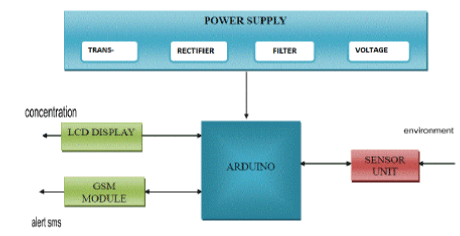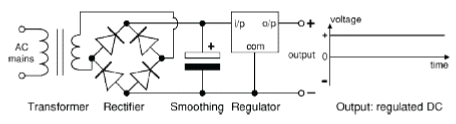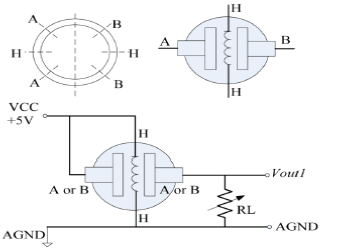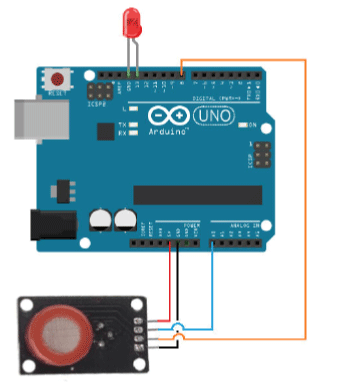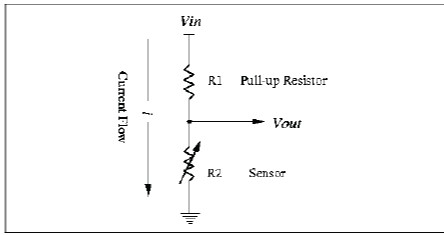Figure 1 Figure 2A Figure 2B Figure 2C Figure 2D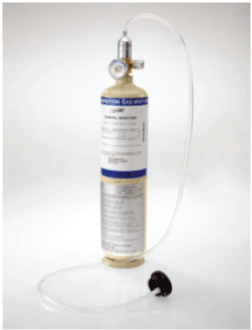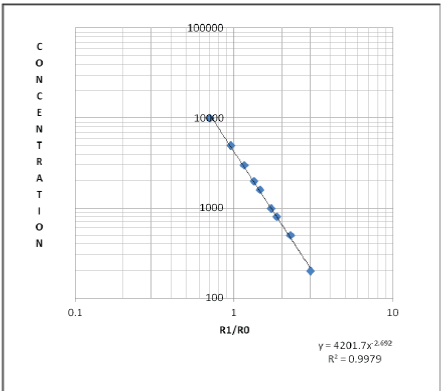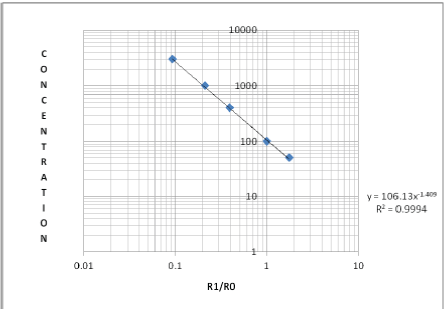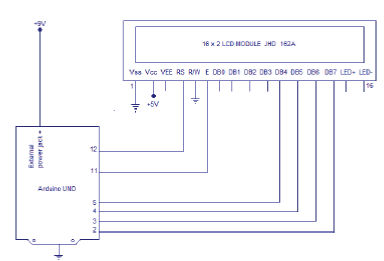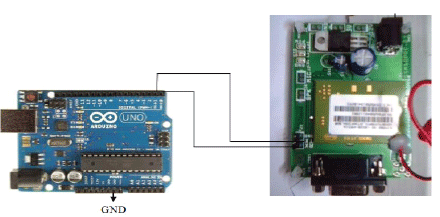Figure 2E Figure 2F Figure 2G Figure 2H Figure 2I